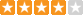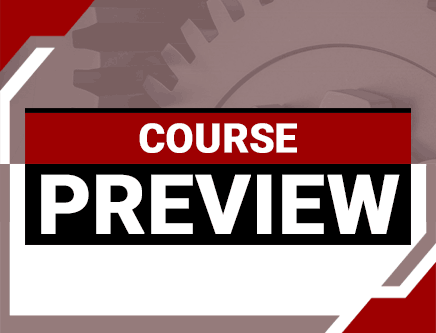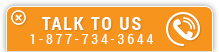Otto Cycle Ideal vs Real Operation Analysis

Course Number: M-1038
Credit: 1 PDH
Subject Matter Expert: Gordan Feric, P.E.
Price: \$29.95 Purchase using Reward Tokens. Details1 reviews
Overview

In Otto Cycle Ideal vs Real Operation Analysis, you'll learn ...

• Understand basic energy conversion engineering assumptions and equations
• Know basic components of the Otto Cycle and its p - V and T - s diagrams
• Be familiar with the Otto Cycle ideal vs. real operation
• Understand general Otto Cycle performance trends

OverviewPreview a portion of this course before purchasing it.

Credit: 1 PDH

Length: 18 pages

In this one hour course, the open, simple Otto Cycle used for stationary power generation is considered.

The Otto Cycle thermal efficiency is presented only for the air as the working fluid. The thermal efficiency derivation is presented with a simple mathematical approach. The Otto Cycle is presented in the p - V and T - s diagrams and its major performance trends (thermal efficiency and power output) are plotted in a few figures as a function of compression ratio, combustor outlet temperature, some fixed cylinder geometry and both isentropic compression and expansion efficiency. It should be noted that this online course does not deal with costs (capital, operational or maintenance).

In this course, the student gets familiar with the Otto Cycle, its components, p - V and T - s diagrams, ideal and real operation and major performance trends.

Specific Knowledge or Skill Obtained

This course teaches the following specific knowledge and skills:

• Understand basic energy conversion engineering assumptions and equations
• Know basic components of the Otto Cycle and its p - V and T - s diagrams
• Be familiar with the Otto Cycle ideal vs. real operation
• Understand general Otto Cycle performance trends

Certificate of Completion

You will be able to immediately print a certificate of completion after passing a multiple-choice quiz consisting of 10 questions. PDH credits are not awarded until the course is completed and quiz is passed.

Board Acceptance
 This course is applicable to professional engineers in: Alabama (P.E.) Alaska (P.E.) Arkansas (P.E.) Delaware (P.E.) Florida (P.E. Area of Practice) Georgia (P.E.) Idaho (P.E.) Illinois (P.E.) Illinois (S.E.) Indiana (P.E.) Iowa (P.E.) Kansas (P.E.) Kentucky (P.E.) Louisiana (P.E.) Maine (P.E.) Maryland (P.E.) Michigan (P.E.) Minnesota (P.E.) Mississippi (P.E.) Missouri (P.E.) Montana (P.E.) Nebraska (P.E.) Nevada (P.E.) New Hampshire (P.E.) New Jersey (P.E.) New Mexico (P.E.) New York (P.E.) North Carolina (P.E.) North Dakota (P.E.) Ohio (P.E. Self-Paced) Oklahoma (P.E.) Oregon (P.E.) Pennsylvania (P.E.) South Carolina (P.E.) South Dakota (P.E.) Tennessee (P.E.) Texas (P.E.) Utah (P.E.) Vermont (P.E.) Virginia (P.E.) West Virginia (P.E.) Wisconsin (P.E.) Wyoming (P.E.)
Reviews (1)
More DetailsPreview a portion of this course before purchasing it.

Credit: 1 PDH

Length: 18 pages